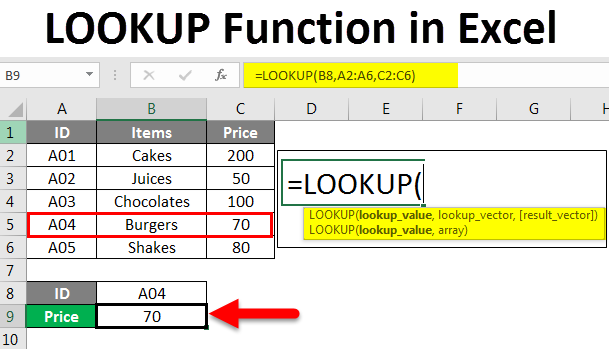Blog

# How to Use Excel Lookup Function?

Are you an Excel user? Do you want to learn how to use the Lookup function to find the right data faster? Lookup functions are an invaluable tool for organizing and accessing data in Excel. With a few simple steps, you can use the Lookup function to get the information you need in a fraction of the time. In this guide, we’ll walk you through how to use the Lookup function in Excel, so you can make the most of your data.## Top 6 Frequently Asked Questions

### What is Excel Lookup Function?

Excel Lookup Function is a function used to search for a certain value within a range of data. It can be used to search for a specific value in a single row or column, or multiple rows and columns. It can also be used to search for a specific value within a given range of data. The Lookup Function can be used for a variety of purposes, including finding the value of a certain cell, looking up data from a table or list, and even finding the position of an item in a list.

### What is the Syntax for Excel Lookup Function?

The syntax for Excel Lookup Function is: =LOOKUP(value, range, ). The “value” is the value that you want to look up. The “range” is the range of cells that will be searched for the value. The optional “result_range” is the range of cells that will be returned if the value is found in the range.

### How do I Use Excel Lookup Function?

Using Excel Lookup Function is relatively straightforward. First, select the cell where you want the result to be returned. Then, type in the formula, =LOOKUP(value, range, ), and hit enter. The result will be the value if it is found in the range, or an error message if it is not found.

### What are the Different Types of Lookup Functions?

There are two different types of Lookup Functions: VLOOKUP and HLOOKUP. VLOOKUP stands for Vertical Lookup and is used to search for a value in a single row or column. HLOOKUP stands for Horizontal Lookup and is used to search for a value in multiple rows or columns.

### What is the Difference Between VLOOKUP and HLOOKUP?

The main difference between VLOOKUP and HLOOKUP is the direction in which they search. VLOOKUP searches vertically, from top to bottom, while HLOOKUP searches horizontally, from left to right. VLOOKUP searches for a single value, while HLOOKUP searches for multiple values.

### When Should I Use Excel Lookup Function?

Excel Lookup Function should be used when you want to quickly search for a specific value within a given range of data. It can be used for a variety of purposes, including looking up data from a table or list, finding the value of a certain cell, and finding the position of an item in a list.

In conclusion, the Excel Lookup function is a powerful tool that can be used to quickly and accurately locate information in a spreadsheet. It is versatile and easy to use, allowing users to save time when searching for specific data. With a few simple steps, you can quickly and easily learn how to leverage the Excel Lookup function to your advantage. Whether you are a beginner or an advanced user, the Excel Lookup function can be a valuable asset in your spreadsheet toolkit.